开关电源的8处损耗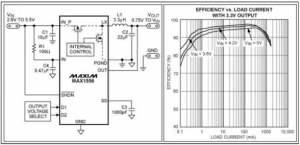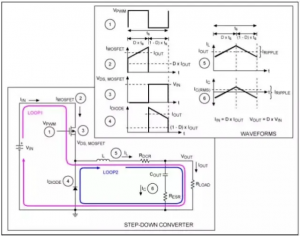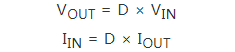1、开关器件的损耗 MOSFET 传导损耗

MOSFET 和二极管是开关元件，导通时电流流过回路。器件导通时，传导损耗分别由MOSFET 的导通电阻(RDS(ON))和二极管的正向导通电压决定。
MOSFET 的传导损耗(PCOND(MOSFET))近似等于导通电阻RDS(ON)、占空比(D)和导通时MOSFET 的平均电流(IMOSFET(AVG))的乘积。
PCOND(MOSFET) (使用平均电流) = IMOSFET(AVG)² × RDS(ON) × D

PCOND(MOSFET) = [(IP3 – IV3)/3] × RDS(ON) × D
= [(IP3 – IV3)/3] × RDS(ON) × VOUT/VIN

PCOND(MOSFET) (使用平均电流) = 12 × 0.1 × 0.5 = 0.050W

PCOND(MOSFET) (使用电流波形积分进行计算) = [(1.753 – 0.253)/3] × 0.1 × 0.5 = 0.089W

2、二极管传导损耗
MOSFET 的传导损耗与RDS(ON)成正比，二极管的传导损耗则在很大程度上取决于正向导通电压(VF)。二极管通常比MOSFET 损耗更大，二极管损耗与正向电流、VF 和导通时间成正比。由于MOSFET 断开时二极管导通，二极管的传导损耗(PCOND(DIODE))近似为：
PCOND(DIODE) = IDIODE(ON) × VF × (1 – D)

PCOND(DIODE) = IOUT × VF × (1 – VOUT/VIN)

3、开关动态损耗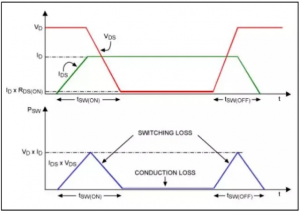PSW(MOSFET) = 0.5 × VD × ID × (tSW(ON) + tSW(OFF)) × fS

PT(MOSFET) = PCOND(MOSFET) + PSW(MOSFET)
= [(I13 – I03)/3] × RDS(ON) × VOUT/VIN + 0.5 × VIN × IOUT × (tSW(ON) + tSW(OFF)) × fS
= [(13 – 03)/3] × 0.1 × 3.3/10 + 0.5 × 10 × 0.5 × (38 × 10-9) × 1 × 106
= 0.011 + 0.095 = 106mW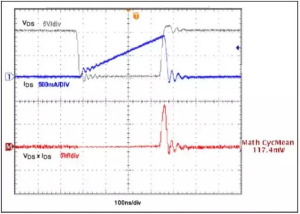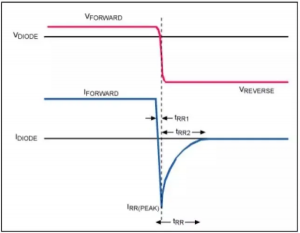PSW(DIODE) = 0.5 × VREVERSE × IRR(PEAK) × tRR2 × fS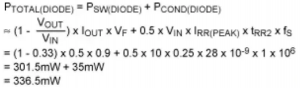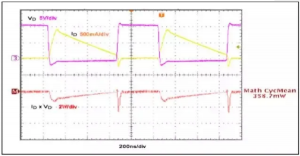4、集成功率开关

SMPS 的控制架构是影响开关电源效率的关键因素之一。这一点我们已经在同步整流架构中讨论过，由于采用低导通电阻的MOSFET 取代了功耗较大的开关二极管，可有效改善效率指标。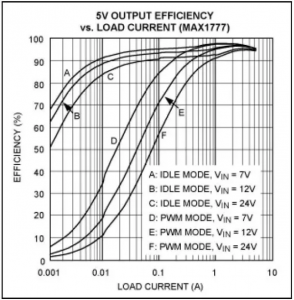5、无源元件损耗

6、电感功耗阻性损耗

DCR 定义为以下电阻公式：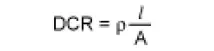DCR 将随着线圈长度的增大而增大，随着线圈横截面积的增大而减小。可以利用该原则判断标准电感，确定所要求的不同电感值和尺寸。对一个固定的电感值，电感尺寸较小时，为了保持相同匝数必须减小线圈的横截面积，因此导致DCR 增大；对于给定的电感尺寸，小电感值通常对应于小的DCR，因为较少的线圈数减少了线圈长度，可以使用线径较粗的导线。

PL(DCR) = LAVG2× DCR

PL(DCR) = (IP3 – IV3)/3 × DCR

7、磁芯损耗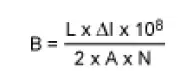8、电容损耗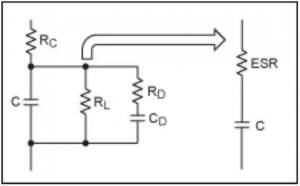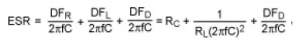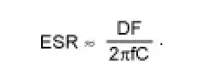PCAP(ESR) = ICAP(RMS)2 × RESR

ICIN(RMS) = IOUT/VIN × [VOUT (VIN – VOUT)]1/2